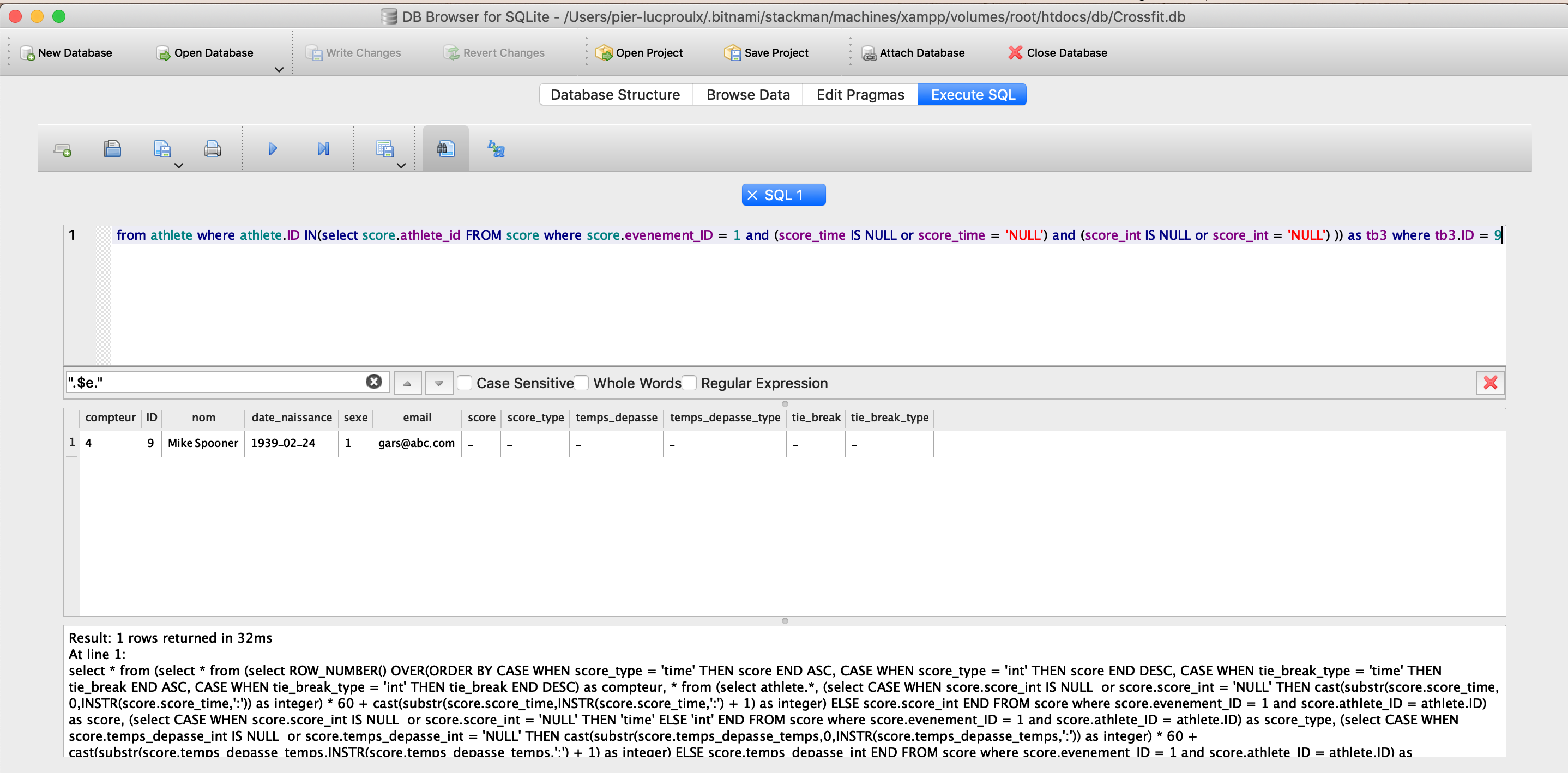# PHP PDO for SQLite Returning false, when clearly DB Browser returns a result-ThrowExceptions

Exception or error:

I’ve been a PHP developper for over 7 years and I’ve never been faced with such an ugly problem. I have a request here, based on SQLite, which returns a result in SQLite, but when I try to put it in a PDO prepared request, it doesn’t return anything, just that there’s a mistake near ‘(‘, which is impossible since DB Browser is clearly returning a result… HELP!

It looks like the request is too long? (See image below, clearly returning a result

See imageCode :

``````try{
\$requete = \$connexion->prepare("select * from (select * from (select ROW_NUMBER() OVER(ORDER BY CASE WHEN score_type = 'time' THEN score END ASC, CASE WHEN score_type = 'int' THEN score END DESC, CASE WHEN tie_break_type = 'time' THEN tie_break END ASC, CASE WHEN tie_break_type = 'int' THEN tie_break END DESC) as compteur, * from (select athlete.*, (select CASE WHEN score.score_int IS NULL or score.score_int = 'NULL' THEN cast(substr(score.score_time,0,INSTR(score.score_time,':')) as integer) * 60 + cast(substr(score.score_time,INSTR(score.score_time,':') + 1) as integer) ELSE score.score_int END FROM score where score.evenement_ID = 1 and score.athlete_ID = athlete.ID) as score, (select CASE WHEN score.score_int IS NULL or score.score_int = 'NULL' THEN 'time' ELSE 'int' END FROM score where score.evenement_ID = 1 and score.athlete_ID = athlete.ID) as score_type, (select CASE WHEN score.temps_depasse_int IS NULL or score.temps_depasse_int = 'NULL' THEN cast(substr(score.temps_depasse_temps,0,INSTR(score.temps_depasse_temps,':')) as integer) * 60 + cast(substr(score.temps_depasse_temps,INSTR(score.temps_depasse_temps,':') + 1) as integer) ELSE score.temps_depasse_int END FROM score where score.evenement_ID = 1 and score.athlete_ID = athlete.ID) as temps_depasse, (select CASE WHEN score.temps_depasse_int IS NULL or score.temps_depasse_int = 'NULL' THEN 'time' ELSE 'int' END FROM score where score.evenement_ID = 1 and score.athlete_ID = athlete.ID) as temps_depasse_type,(select CASE WHEN score.tie_break_int IS NULL or score.tie_break_int = 'NULL' THEN cast(substr(score.tie_break_time,0,INSTR(score.tie_break_time,':')) as integer) * 60 + cast(substr(score.tie_break_time,INSTR(score.tie_break_time,':') + 1) as integer) ELSE score.tie_break_int END FROM score where score.evenement_ID = 1 and score.athlete_ID = athlete.ID) as tie_break,(select CASE WHEN score.tie_break_int IS NULL or score.tie_break_int = 'NULL' THEN 'time' ELSE 'int' END FROM score where score.evenement_ID = 1 and score.athlete_ID = athlete.ID) as tie_break_type from athlete) as tb where score and score > 0 is not null ORDER BY CASE WHEN score_type = 'time' THEN score END ASC, CASE WHEN score_type = 'int' THEN score END DESC, CASE WHEN tie_break_type = 'time' THEN tie_break END ASC, CASE WHEN tie_break_type = 'int' THEN tie_break END DESC) as tb2 UNION select (select COUNT(*) from score where score.evenement_ID = 1 ) - (select COUNT(*) from score where (score_int is null or score_int = 'NULL') and (score_time is null or score_time = 'NULL') and score.evenement_ID = 1 ) + 1 as compteur, athlete.*,'-' as score_int,'-' as score_type,'-' as temps_depasse ,'-' as temps_depasse_type,'-' as tie_break , '-' as tie_break_type from athlete where athlete.ID IN(select score.athlete_id FROM score where score.evenement_ID = 1 and (score_time IS NULL or score_time = 'NULL') and (score_int IS NULL or score_int = 'NULL'))) as tb3 where tb3.ID = 9"
);
\$requete->execute();
\$tableau_personne = \$requete->fetch();

}catch(PDOException \$w){
echo("OK");
echo(\$w->getMessage());
}
``````

This is the error that PHP is returning :

Warning: PDO::query(): SQLSTATE[HY000]: General error: 1 near “(“: syntax error in /opt/lampp/htdocs/application/competition.php on line 190
Array (  => HY000  => 1  => near “(“: syntax error )
Fatal error: Uncaught Error: Call to a member function execute() on bool in /opt/lampp/htdocs/application/competition.php:194 Stack trace: #0 {main} thrown in /opt/lampp/htdocs/application/competition.php on line 194

How to solve: